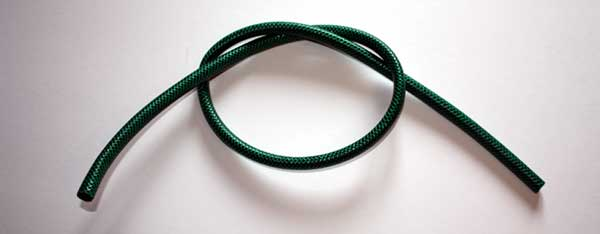Basic principles of Electricity

This page is about why current flows in a wire and Ohms law, check out the main index for the rest.

Imagine a hose pipe:A tap is connected to one end of the hose, water flows through the hose and out of the other end. This is because one end of the hose has at a higher pressure to the other. If we restrict the water flow by squeezing the hose pipe then less water will flow.

This is the same with an electric circuit. We must have a difference in ‘pressure’ from one point in the circuit to another for electric current to flow. This is called potential difference and it is measured in volts. The flow of electricity is calledcurrent and it is measured in amps. The resistance to electricity is measured in Ohms.This is why a battery has two terminals and we must make a complete circuit for current to flow – one terminal is a higher pressure than the other, so there is a difference in pressure between them.

Potential difference, measured in volts, is equivalent to water pressure in the hose.

Current measured, in amps, is equivalent to water flowing in the hose.

Squeezing or obstructing the water flow in the hose is resistance, measured in Ohms.

Potential difference, current and resistance are all related by Ohms law, so we can calculate any of the numbers by transposing the formula. V stand for Volts, I stands for Current, R stands for Resistance.

V = IR

I = V/R

R = V/I

Therefore if the bulb in our circuit has a resistance of 100 Ohms, our potential difference is 12 volts, then the current flow is:

I = 12/100

I = 0.12 Amps or 120 mA (milliamps)